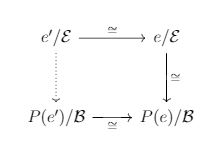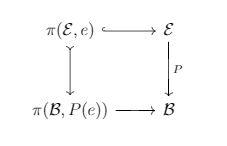# Model Category For Groupoids

Published:

$\newcommand{\rv}{\mathbf{#1}} \newcommand{\x}{\rv x} \newcommand{\y}{\rv y} \newcommand{\bar}{\overline{#1}} \newcommand{\wtil}{\widetilde{#1}} \newcommand{\what}{\widehat{#1}} \newcommand{\ep}{\varepsilon} \newcommand{\ph}{\varphi} \newcommand{\maps}{\colon} \newcommand{\to}{\rightarrow} \newcommand{\xraw}{\xrightarrow} \newcommand{\darr}{\downarrow} \newcommand{\To}{\Rightarrow} \renewcommand{\dot}{\centerdot} \renewcommand{\tensor}{\otimes} \newcommand{\pr}{^\prime} \newcommand{\op}{#1^{\mathrm{op}}} \newcommand{\hom}{\textit{Hom}} \newcommand{\oo}{\circ} \newcommand{\lang}{\langle} \newcommand{\rang}{\rangle} \newcommand{\str}{#1^{*}} \newcommand{\intvl}{\mathbb{I}} \newcommand{\yon}{\mathcal{Y}} \newcommand{\topos}{\mathfrak{Top}} \newcommand{\bTopos}{\mathfrak{BTop}} \newcommand{\BTop}{\mathfrak{BTop}} \newcommand{\con}{\mathfrak{Con}} \newcommand{\pos}{\mathfrak{Poset}} \newcommand{\topl}{\mathbb{Top}} \newcommand{\grpd}{\mathbb{Grpd}} \newcommand{\ab}{\mathbf{Ab}} \newcommand{\thT}{\mathbb{T}} \newcommand{\mod}{\mathbf{Mod}} \newcommand{\th}{\mathbf{Th}} \newcommand{\cl}{\mathbf{Cl}} \newcommand{\ob}{\mathbf{Ob}} \newcommand{\aut}{\mathbf{Aut}} \newcommand{\bun}{\mathbf{Bun}} \newcommand{\geom}{\mathbf{Geom}} \def\catg{\mathop{\mathcal{C}\! {\it at}}\nolimits} \def\Cat{\mathop{\mathfrak{Cat}}} \def\Con{\mathop{\mathfrak{Con}}} \def\CAT{\mathop{\mathbf{2} \mathfrak{Cat}}} \def\Cat{\mathop{\mathfrak{Cat}}} \newcommand{\Topos}{\mathfrak{Top}} \newcommand{\ETopos}{\mathcal{E}\mathfrak{Top}} \newcommand{\BTopos}{\mathcal{B}\mathfrak{Top}} \newcommand{\GTopos}{\mathcal{G}\mathfrak{Top}} \newcommand{\Psh}{\textit{Psh}} \newcommand{\Sh}{\textit{Sh}} \newcommand{\psh}{\textit{Psh}(\cat{#1})} \newcommand{\sh}{\textit{Sh}(\cat{#1})} \newcommand{\Id}{\operatorname{Id}} \newcommand{\Ho}{\operatorname{Ho}} \newcommand{\ad}{\operatorname{ad}} \newcommand{\Adj}{\operatorname{Adj}} \newcommand{\Sym}{\operatorname{Sym}} \newcommand{\Set}{\operatorname{Set}} \newcommand{\Pull}{\operatorname{Pull}} \newcommand{\Push}{\operatorname{Push}} \newcommand{\dom}{\operatorname{dom}} \newcommand{\cod}{\operatorname{cod}} \newcommand{\fun}{\operatorname{Fun}} \newcommand{\colim}{\operatorname{Colim}} \newcommand{\cat}{\mathcal{#1}} \newcommand{\scr}{\mathscr{#1}} \newcommand{\frk}{\mathfrak{#1}} \newcommand{\bb}{\mathbb{#1}} \newcommand{\CS}{\mathcal{S}} \newcommand{\CE}{\mathcal{E}} \newcommand{\CF}{\mathcal{F}}$

Warning: These notes are severely incomplete! Hopefully I will finish them soon.

Abstract:

## The fundamental groupoid $2$-functor

There is a strict $2$-fucntor $\Pi : \topl \to \grpd$ which associates to every topological space its fundamental groupoid, to a continuous map of spaces, a functor of groupoids, and to a homotopy of maps, an iso natural transformation.

For each groupoid $\cat{C}$ and each object $c$ of $\cat{C}$, $\pi(\cat{C}, c)$ is the full subgroupoid of $\cat{C}$ with only one object namely $c$. So, $\pi(\cat{C}, c) (c,c) = \aut_{\cat{C}} (c)$ Composing with $\Pi$, we get the familiar fundamental groups at different points.

We recall from algebraic topology that a continuous map $p:E \to B$ is said to be a covering map (or space $E$ a covering space over $B$) whenever for every $x \in B$ there is an open neighbourhood $U$ containing $x$ such that $p^{-1} (U) = \amalg_{i \in I} V_i$, a disjoint union of open sets $V_i$ in $E$ such that $p|_{V_i}: V_i \cong U$. One handy example of a covering map is the quotient map $\bb{R}^2 \to \bb{T}$ where the torus $\bb{T}$ is obtained as the quotient space of $\bb{R}^2$ by identifying $(x,y) \sim (x+m, y+n)$ for every $m, n \in \bb{Z}$. Another well-known examples is the helix-shaped real line $\bb{R}$ over $1$-sphere $\bb{S}^{1}$.

Remark: If $f : A \to B$ is a map whereby $A$ is path connected then the pullback of $p$ along $f$, that is $\str{f}p$ is a covering map.

There is fundamental lifting type theorem associated to covering maps; the unique path lifting theorem. You can find its proof in section 3.2. of Peter May’s A Concise Course in Algebraic Topology.

Lifting of paths and homotopies written in terms of $2$-functor $\Pi$ yields that $e/P: e/\Pi(E) \to p(e)/\Pi(B)$ is an isomorphism of groupoids for any point $e \in E$, and furthermore the functor $\Pi(p): \Pi(E) \to \Pi(B)$ is surjective on objects. Also, if $\cat{E}=\Pi(E)$ and $\cat{B}=\Pi(B)$, for each object $b$ of $\cat{B}$, the fibre groupoid $\cat{E}_b$ has as objects all points of space $E$ in the pre-image $p^{-1}(b)$ and as morphisms all paths (up to homotopy) between such points which are null-homotopic when composed with $p$: that is all $\lambda: \intvl \to E$ with $p(\lambda (0))=b$ and $p(\lambda(1))=b$ and $p \oo \lambda$ is homotopic to $id_b$.

This motivates us to define the notion of Covering functors of groupoids: For groupoids $\cat{E}$ and $\cat{B}$, a functor $P: \cat{E} \to \cat{B}$ is a covering functor if

1. it is surjective on objects
2. $e/\cat{E} \to P(e)/\cat{B}$ is isomorphism of categories.

Remark: For any groupoid $\cat{E}$, there is only a unique morphism between any two objects of $e/\cat{E}$. So, isomorphism of such co-slice categories means isomorphism of their object sets.

Remark: If the groupoid $\cat{E}$ is connected then it is sufficient to check the second condition of covering only for a single object $e$ of $\cat{E}$. This is because for any other object $e\pr$ there is a morphism $h: e \to e\pr$ in groupoid $\cat{E}$, and the top and bottom functors, defined by precomposition with $h$, are isomorphism as well as the right leg in the following diagram.Also, it follows that for any covering functor of (connected) groupoids, and any $e$ in $\cat{E}$ the induced map of groups is monomorphism: